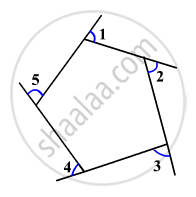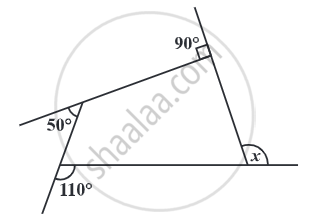# Exterior Angles of a Polygon:

• The exterior angles of a triangle are the angles that form a linear pair with the interior angles by extending the sides of a triangle.

• The sum of the measures of the external angles of any polygon is 360°.

•• The sum of an interior angle and its corresponding exterior angle is always 180 degrees since they lie on the same straight line.
• In the figure, angles 1, 2, 3, 4, and 5 are the exterior angles of the polygon.

# Property of Exterior Angles of a Polygon:

### The sum of the measures of the external angles of any polygon is 360°.We want to know that the total measure of angles, i.e, m∠1 + m∠2 + m∠3 + m∠4 + m∠5.

Let us say you start travelling from the vertex at ∠1. You go in a clockwise direction, make turns through ∠2, ∠3, ∠4, and ∠5 and come back to the same vertex. You covered the entire perimeter of the polygon and in fact, made one complete turn in the process. One complete turn is equal to 360 degrees.

Thus, m∠1 + m∠2 + m∠3 + m∠4 + m∠5 = 360°.
Therefore, the sum of the measures of the external angles of any polygon is 360°.

## Example

Find measure x in the following Fig.x + 90° + 50° + 110° = 360° .....(The sum of the measures of the external angles of any polygon is 360°.)
x + 250° = 360°
x = 110°.

## Example

Find the number of sides of a regular polygon whose each exterior angle has a measure of 45°.
Total measure of all exterior angles = 360°
Measure of each exterior angle = 45°
Therefore, the number of exterior angles = 360/45 = 8
The polygon has 8 sides.
If you would like to contribute notes or other learning material, please submit them using the button below.

### Shaalaa.com

Sum of Measures of External Angles of Triangle [00:04:01]
S
0%